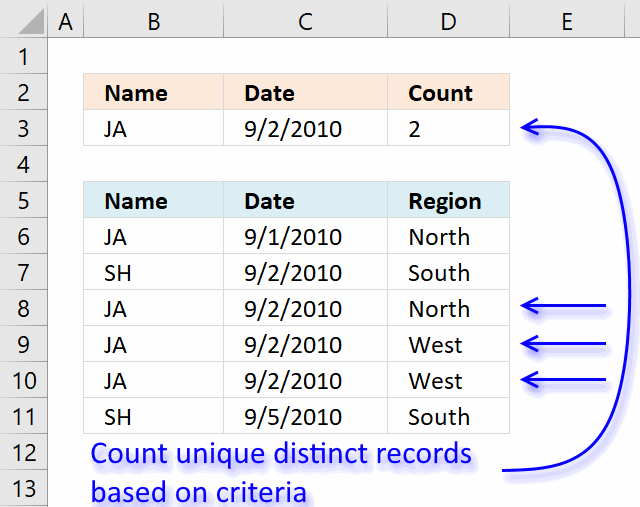Author: Oscar Cronquist Article last updated on January 19, 2019davidlim asks:

re: Count records between two dates and a criterion

based on the example, i was looking for 1 date and 1 criterion. i slightly modify the formula to

=SUMPRODUCT(--(\$B\$1:\$B\$9=\$E\$2), --(\$A\$1:\$A\$9=\$E\$3)) + ENTER

[assuming E2 = 9-2-2010]

the result would be 1 (one 'JA' found on 9-2-2010 date)

but this is summation of records found on 1 date with 1 criterion. It will not work if there is *multiple* 'JA' criterion exist on the same date because SUMPRODUCT summed up the records found.

I'm curious to know...

1) What if I want to know the UNIQUE DISTINCT records found on 1 date with 1 criterion?

2) Working on >100k rows of data, this formula literally slows down Excel (heavy calculation and recalculations). Is there an alternative to speed it up? UDF? array formula?

thanks!

### Answer:

Array formula in cell D3:

=SUM(IF((\$B\$6:\$B\$11=B3)*(\$C\$6:\$C\$11=C3), (1/COUNTIFS(\$B\$6:\$B\$11, \$B\$6:\$B\$11, \$C\$6:\$C\$11, \$C\$6:\$C\$11, \$D\$6:\$D\$11, \$D\$6:\$D\$11)), 0))

### How to create an array formula

1. Copy (Ctrl + c) and paste (Ctrl + v) array formula into formula bar.
2. Press and hold Ctrl + Shift.
3. Press Enter once.
4. Release all keys.

### How the array formula in cell D3 works

Step 1 - Count records

The COUNTIFS function calculates the number of cells across multiple ranges that equals all given conditions.

COUNTIFS(\$B\$6:\$B\$11, \$B\$6:\$B\$11, \$C\$6:\$C\$11, \$C\$6:\$C\$11, \$D\$6:\$D\$11, \$D\$6:\$D\$11)

becomes

COUNTIFS({"JA";"SH";"JA"; "JA";"JA";"SH"}, {"JA";"SH";"JA"; "JA";"JA";"SH"}, {40422;40423; 40423;40423;40423;40426}, {40422;40423; 40423;40423;40423;40426}, {"North";"South";"North"; "West";"West";"South"}, {"North";"South";"North"; "West";"West";"South"})

and returns array {1;1;1;2;2;1}

Step 2 - Filter records using name and date criteria

The IF function returns one value (argument2) if TRUE and another (argument3) if FALSE.

IF((\$B\$6:\$B\$11=B3)*(\$C\$6:\$C\$11=C3), (1/COUNTIFS(\$B\$6:\$B\$11, \$B\$6:\$B\$11, \$C\$6:\$C\$11, \$C\$6:\$C\$11, \$D\$6:\$D\$11, \$D\$6:\$D\$11)), 0)

becomes

IF((\$B\$6:\$B\$11=B3)*(\$C\$6:\$C\$11=C3), (1/{1;1;1;2;2;1}), 0)

becomes

IF(({"JA";"SH";"JA";"JA";"JA";"SH"}="JA")*({40422;40423;40423;40423;40423;40426}=40423), (1/{1;1;1;2;2;1}), 0)

becomes

IF(({0;0;1;1;1;0}, (1/{1;1;1;2;2;1}), 0)

becomes

IF(({0;0;1;1;1;0}, {1;1;1;0,5;0,5;1}, 0)

and returns {0;0;1;0,5;0,5;0}

Step 3 - Sum values

The SUM function adds numbers an return the total.

=SUM(IF((\$B\$6:\$B\$11=B3)*(\$C\$6:\$C\$11=C3), (1/COUNTIFS(\$B\$6:\$B\$11, \$B\$6:\$B\$11, \$C\$6:\$C\$11, \$C\$6:\$C\$11, \$D\$6:\$D\$11, \$D\$6:\$D\$11)), 0))

becomes

=SUM({0;0;1;0,5;0,5;0}) and returns 2 in cell D3.

### Download Excel fileCount-unique-distinct-records-between-two-dates-and-a-condition.xlsx